# Breaking the Equation ' Empirical Argument = Proof '

##### Age 7 to 18

Published 2011 Revised 2021

Empirical argument vs. proof

Consider the generalisation: "the sum of any two odd numbers is an even number." What argument would your students offer for it? Would that be a proof?

An overwhelming body of research shows that students of all levels of schooling including high-attaining secondary students "prove" mathematical generalisations such as the above by using empirical arguments (e.g., Coe and Ruthven, 1994). By empirical arguments I mean those that purport to show the truth of a generalisation by validating the generalisation in a proper subset of all possible cases. These arguments are clearly invalid, because they cannot exclude the possibility of the existence of a counterexample to the generalisation. Here are two examples of empirical arguments for the above generalisation:

Empirical argument 1: naive empiricism

I tried many different pairs of odd numbers and their sum was always an even number: $\bf 7 + 9 = 16$, $\bf 15 + 21 = 36$, $\bf 25 + 27 = 52$, etc. So the sum of any two odd numbers is an even number.

Empirical argument 2: crucial experiment

I checked different kinds of pairs of odd numbers: some with small odd numbers (e.g., $1 + 9 = 10$), some with big odd numbers (e.g., $213 + 399 = 612$), some with the same odd numbers (e.g., $25 + 25 = 50$), and some with prime odd numbers (e.g., $17 + 31 = 48$). No pair gave me a counterexample - the sum was always an even number. So the sum of any two odd numbers is an even number.

Even though both arguments are invalid, the second argument can be considered more advanced than the first, because, by seeking possible counterexamples, it communicates a concern that the generalisation may not be true. Balacheff (1998) used the terms naive empiricism and crucial experiment to describe the special categories of empirical arguments represented by the first and second examples, respectively. The search of possible counterexamples in crucial experiment requires a strategic selection of cases in contrast to the random (or convenience) sampling of cases in naive empiricism.

The fact that a generalisation is true in some cases does not guarantee and, thus, does not prove that the generalisation is true for all possible cases. This is the main limitation of any kind of kind of empirical argument that many students find difficult to understand. What would be a proof for the generalisation then? Figure 1 shows three possible proofs for the generalisation on the set of whole numbers.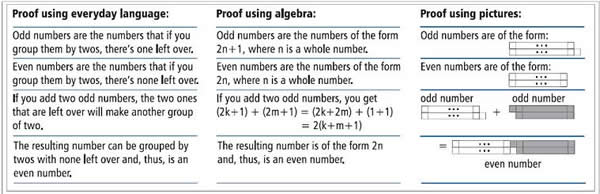Figure 1: Three possible proofs (on the set of whole numbers) for 'odd + odd = even.'

Notice the correspondences among the three arguments: they all seem to be saying the 'same thing' using different representations. Notice also how each argument can be used to help someone understand why the generalisation is true, but also convince someone that the generalisation is true for all cases without requiring that person to make a leap of faith. A proof's potential to promote understanding and conviction is one of the main reasons why proof is so important for students' learning of mathematics.

A question that arises at this point is: How can we help students overcome the misconception that 'empirical argument = proof '? Unless students realise the limitations of empirical arguments as methods for validating mathematical generalisations, they are unlikely to appreciate the importance of proof in mathematics.

Next I describe and discuss a mathematics lesson in a high-attaining Year 10 class that aimed to help the students begin to realise the limitations of empirical arguments. The lesson was an adapted version of one developed by a research project in the context of a university course (Stylianides and Stylianides, accepted). I worked with the teacher of the Year 10 class and another Year 10 teacher in the same school to adapt the lesson to the particular context of their two classes, and then I observed the lesson being taught in each class. The lesson plan, in the form of annotated PowerPoint slides, is available at www.atm.org.uk/mt213.

The lesson

The lesson was approximately 60 minutes long and was taught over two consecutive 45-minute periods. The lesson involved three activities: the Squares Problem, the Circle and Spots Problem, and the 'Monstrous Counterexample'. As you read the following sections, I invite you to pay attention to how each activity was used by the teacher to facilitate students' progression along the 'learning path' in Figure 2: from using naive empiricism as a method for validating patterns, to using crucial experiment, to feeling a need to learn about more secure methods for validating patterns (i.e., to learn about proofs). Note that a pattern is a kind of generalisation. The teacher and student names are pseudonyms.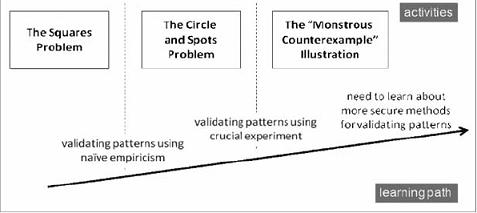Figure 2: The three activities and corresponding 'learning path.'

Activity 1: The squares problem

Kathy, the teacher, introduced the Squares Problem (Figure 3). The hardest part of the problem was the third: it asked students to find the number of different 3-by-3 squares in a case that was difficult for them to check practically and also to explain whether and why they were sure their answer was correct.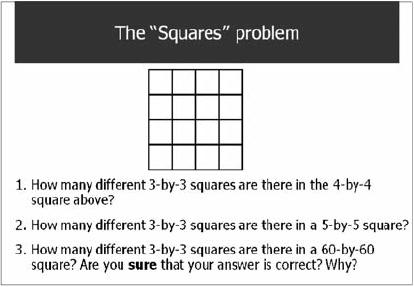Figure 3: The Squares Problem (adapted from Zack, 1997).

Kathy made sure the students understood what the problem was saying and then she asked them to work on the problem in their small groups. The small group closest to myself had six students: Bob, Calvin, Dan, Lazarus, Robert, and Sharon. These students counted squares to answer parts 1 and 2 of the problem, and then Bob asked his peers: "Have you actually got a formula?" Dan responded: "It's the number of ... it's $n$ minus $2$, and then squared." Sharon showed excitement and confirmed with Dan that the answer for part 1 would be $4$. Robert asked how many 3-by-3 squares there were in a 60-by-60 square (part 3) and Dan used his calculator and the formula he had described earlier to find the answer: $(60 - 2)^2 = 3364$.

At some point Kathy visited the small group and the students explained their work. Kathy then asked the students whether they were sure their answer was correct. Lazarus replied "yes" with confidence and Kathy posed a new question: "And have you thought about why you are sure?" There was no response from the students. Kathy asked the students to think about this and write their ideas on paper.

Dan drew figures for the 4-by-4 and 5-by-5 squares showing the 3-by-3 squares in each of them. He wrote down $58^2 = 3364$ as the answer to part 3 and also the formula $(n - 2)^2$. He concluded: "We realised that if you took 2 away from the number of cubes along the top and then square the answer you will get the number of 3$\times$3 boxes in the grid.?" The other students in the small group wrote similar conclusions in their papers.

So, what has happened thus far in the small group? The students identified the pattern that the number of different 3-by-3 squares in an n-by-n square was given by the formula $(n - 2)^2$. They verified the pattern for $n=4$ and $n=5$ and, based on these results, they concluded that the pattern would hold true for all values of $n$ including $n=60$. Thus the students validated the pattern on the basis of naive empiricism (cf. Figure 2).

The whole group discussion that followed illustrated further the use of naive empiricism in the class, as all groups answered the three parts of the problem using the formula $(n - 2)^2$. After some discussion on the meaning of the formula, Kathy asked the class whether and why they could be sure that their answers based on this formula were correct. Emily said: "We tried it [the formula] for a 6-by-6 square and it worked for that too. " Kathy invited further comments but the students did not have anything to add to what Emily had said.

Kathy then asked the students to write down individually their thoughts: "I want to know what your feelings are about whether this [the answer to part 3] is correct or not. You may think it is correct, you may not. If you are sure, I want to learn why you are sure." Someone asked "what if you're not sure?" and Kathy responded "then put not sure, but say why you are not sure - what makes you doubt it?"

In the focal small group the students wrote:
• Bob: "Because we have found a formula and tried it against smaller squares so we can make sure that the formula is right."
• Calvin: "I am sure that this solution works because it worked for every one we did."
• Dan: "I am sure that the answer is correct because it has been proved for a number of smaller grids."
• Lazarus: "I am sure that the answer is correct because it has been tested and proved correct. The pattern will continue to $60\times60$."
• Robert: "I am sure it's correct because we did a test on the $6 \times 6$ grid and it worked."
• Sharon: "We are sure that it is right because we have tried it for a $6 \times 6$ square as well. So we assume that it would work."

Notice that the six students were convinced of the truth of the pattern on the basis of naive empiricism: the pattern worked for the first few cases and so, according to the students, it would work also for $n=60$. This reasoning was reflected in the writings of the rest of the class, something that we had anticipated in our planning and Kathy confirmed as she was circulating around and looking at students' papers.

Following the students' individual reflections, Kathy proceeded with the next item in the lesson plan, which was to summarise students' validation method thus far:

"I get a feeling that most of you have said 'Well, I think we have sort of answered this question that $58^2$ is the right answer: we have found a pattern by checking smaller grid sizes and then we have used that pattern, assuming that it would continue all the way up to 60-by- 60.' That's the stage where we are right now: we've seen a pattern working, somebody said they tried the 6-by-6 and it worked for that too, and so we continued our pattern up to the $58^2$."

Bob asked Kathy whether the pattern was correct and Kathy said that the class would come back to this issue later, but first they would work on a couple of other activities. Indeed, according to our lesson plan the issue about the correctness of the pattern in the Squares Problem would remain tentatively unresolved. The class would revisit and resolve the issue after the students had been assisted to realise the limitations of empirical arguments (both naive empiricism and crucial experiment). Had the issue been resolved at this point of the lesson, this would probably require a lot of 'telling' by the teacher, which was inconsistent with our goals in the lesson. We wanted the students to realise the limitations of empirical arguments on their own, by experiencing and reflecting on situations where the empirical validation method was inadequate. For the readers' information, I note that the $(n - 2)^2$ pattern was actually correct.

Activity 2: The circle and spots problem

Kathy introduced the Circle and Spots Problem (Figure 4) and helped the students understand what the problem was saying. Specifically, she discussed with them the meaning of the terms 'maximum' and 'non-overlapping regions' Also, she clarified that the phrase 'around the circle' referred to the circle's circumference and that the spots on the circumference did not have to be equidistant. Then Kathy asked the students to work on the problem in their small groups.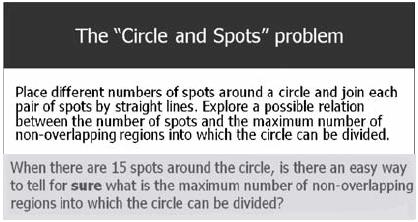Figure 4: The Circle and Spots Problem (adapted from Mason et al, 1982).

Notice that, similar to part 3 of the Squares Problem, the question in the Circle and Spots Problem (pale grey box in Figure 4) was asking the students to make a statement about a case that was difficult for them to check practically. In our planning we had anticipated that the students, like they did in the Squares Problem, would check simpler cases, identify a pattern, trust the pattern based on naive empiricism, and apply it to offer a definite answer for $n=15$ (where $n$ stands for the number of spots). The main difference between the two problems is that the emerging pattern in the Circle and Spots Problem fails for $n=6$. Our plan was for Kathy to use the anticipated surprise that the students would experience with the failing pattern to help them move from naive empiricism towards crucial experiment (cf. Figure 2).

After about 10 minutes of small group work, Kathy brought the whole class together and said: "Circulating around I think there are some people who think they know what the answer will be for 15 [spots]. Is there anyone who is willing to tell us their number of regions, what it will be for 15 spots?"

Mac said that his group thought the formula for the problem was $(n - 1)^2$ but soon thereafter he corrected himself to say the formula included powers of $2$. Kathy asked the class to say the maximum number of non-overlapping regions they found for different spots, and she constructed a table on the board with the following numbers: $4$, $8$, and $16$, for $n = 3$, $4$, and $5$, respectively. Then she pointed out that, as Mac had mentioned earlier, the values were all powers of $2$ and that, in each case, the power was one less than the number of spots: $2^2$ (for $n=3$), $2^3$ (for $n=4$), and $2^4$ (for $n=5$). Kathy asked: "So what will it be for 15 spots then?"

Several students offered to answer Kathy's question. Based on what I had observed during these students' prior work in their small groups, I presumed they would propose the application of the $2^{n-1}$ formula for $n=15$. However, Ken said loudly: "Can I just say that is wrong because on $6$ [spots] there are only $30$ [regions]." Kathy said: "We were about to say that the answer would be $2$ to the power of $14$. However, you are telling me that for $6$ spots it doesn't work out to be... With this pattern for $6$ six spots it would be $2$ to the power of $5$, that would be $32$, but did anyone manage to find this number of spots?" Some students said they found $31$ spots.

Kathy continued:

"When we were back to the Squares Problem, we said that because the pattern worked for some of the different grids, the 5-by-5, 6-by-6 squares, and so on, we were willing to trust it. But this time we have shown that it works for $3$, it works for $4$, it works for $5$, but actually, Ken, you are right: if we had $6$ spots on a circle and we joined them all up, the number of nonoverlapping regions that we get is not what we expect to get, it's not $32$. It's actually $31$."

As she talked, Kathy used a PowerPoint slide to illustrate the counterexample for $n=6$. She noted also that, if one drew the spots in a regular hexagon, the maximum number of regions would be $30$, which is again smaller than $32$. Then, following the lesson plan, Kathy asked the students to write down their thoughts about what the Circle and Spots problem had taught them.

The students in the focal small group wrote:

• Bob: "You can't always trust a formula until you have tested it many times over for lots of different examples."
• Calvin: "This test has taught us that if you see a pattern doesn't make it correct."
• Dan: "The circle and spots tells us that we can't always trust a formula that works on the first few."
• Lazarus: "This teaches us that just because something works for one thing, that doesn't mean it will work for everything."
• Robert: "You can't always trust a formula until you have tested many times over for lots of different numbers of spots."
• Sharon: "You can't always trust a formula. You shouldn't presume it is correct because it worked for the first few."

Notice that the students began to move away from naive empiricism. For example, Dan, Lazarus, and Sharon started feeling uneasy to trust a pattern based on checks of the first few cases. Also, Bob and Robert's comments approximated the crucial experiment method of validation, as they appeared to raise a concern about the number ('many') and quality ('different') of cases that had to be checked before a pattern could be trusted.

Thus an important issue for many students at this stage of the lesson was how many cases would be enough for them to check before trusting a pattern. We had anticipated this issue in our planning and we prepared a PowerPoint slide with a fictional student comment on it that Kathy used in the lesson to organise a discussion around the issue. The fictional student comment said:

"The Circle and Spots Problem teaches me that checking $5$ cases is not enough to trust a pattern in a problem. Next time I work with a pattern problem, I'll check more cases to be sure."

Kathy invited reactions from her students on this comment. Dan suggested trying spread cases such as for $n = 1$, $75$, and $100$. Robert observed that "you can't always trust the formula, you have to test it." Kathy asked Robert how many times one had to test a formula and Robert said "more than like 5 times." Kathy invited more comments and Larry said: "you should test it as many times as you have time to do." Kathy asked Larry: "So when you have tested it as many times as you have time to do, can you then trust it?" Larry revised: "No ... not a 100%!" Then Pauline said: "try it out with smaller numbers and bigger numbers." Kathy observed that Pauline's comment was similar to Dan's earlier comment.

Indeed, the two comments were similar to one another and illustrative of the crucial experiment method for validating patterns (cf. Figure 2). As I noted earlier, crucial experiment can be considered to be a more advanced method than naive empiricism, but is still an invalid, for a counterexample may exist in a case that was not checked. Some students in the class were thinking along similar lines, as illustrated by their responses to Kathy's question: "And then do we trust it if it worked for all of those [cases, big and small ones]?" Silvia said in a low voice: "No, because you might have missed one." Another student was heard to say: "You could spend your whole life and still miss one!" These students' fear that a pattern can fail in a case that was not checked was manifested in the next activity we planned for the students.

Activity 3: The 'Monstrous Counterexample' illustration

Kathy introduced the PowerPoint slide in Figure 5 that shows what I call the 'Monstrous Counterexample' Illustration. Kathy did not use this name during the lesson. The slide was presented in segments to give students a chance to process the information in it. For example, there was a discussion about how one would check whether a given number was a square number using a calculator. Also, the students confirmed the statement for particular values of $n$ using their calculators.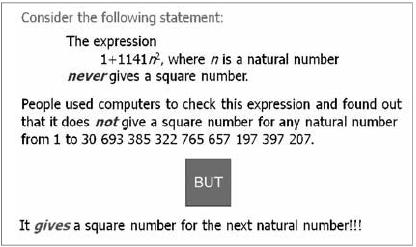Figure 5: The 'Monstrous Counterexample' Illustration (adapted from Davis,1981).

Once the students checked many different cases and were comfortable with the meaning of the statement, Kathy presented the counterexample. The students were amazed: they had not anticipated that a pattern that held for so many cases (of the order of septillions) could ultimately fail!

Kathy then directed the students' attention to their previous discussion: "We said in the Circle and Spots Problem that, okay, it's not enough to just check a few cases, you need to try different ones. Well, this expression, what does this tell us?" Emily said: "If you kept trying, you might have to go that high until you find one [a counterexample]." Kathy said: "But I can imagine that it took the computer quite a long time to check all of those cases. And when do you stop checking?" Larry said: "when you've found one!" Several students laughed with what Larry had said. Kathy continued: "And when do you trust a pattern then?" Adam said: "When you cannot find one, until you are dead!"

Notice that the students began to develop distrust in empirical arguments of any kind, including crucial experiment. Yet, although the students began to realise the limitations of empirical arguments, they lacked knowledge of more secure methods for validating patterns. This caused a feeling of frustration among some of them as illustrated in Adam's comment: one would die checking cases before being in a position to trust a pattern! Thus we may say that the students reached the point when they felt a need to learn about more secure validation methods (cf. Figure 2).

The misconception that 'empirical arguments = proofs' is deeply rooted in many students' thinking. Nevertheless, the story I presented in this article sends the optimistic message that it is possible to help students realise the limitations of empirical arguments and create a need in them to learn about more secure methods for validating patterns. Needless to say, it is not enough for teachers to create this need in students and then leave them in a state of frustration. Teachers have the responsibility to also help their students appreciate the role of proof as a secure method for validating patterns in mathematics, to teach them what is involved in developing a proof, and give them opportunities to develop and criticise proofs against a list of criteria that students can understand. This is precisely what happened in subsequent lessons in Kathy's class: she introduced her students to the notion of proof in mathematics and she took them back to the Squares Problem and helped them develop a proof for the pattern they had identified earlier. The next part of the story will appear in a future article!

Andreas J. Stylianides
Article taken from Mathematics Teaching 213 / March 2009

Trained as a primary teacher in Cyprus, Andreas Stylianides studied for a masters in maths education, as well as a masters in mathematics, in the United States. He followed these studies with a PhD in mathematics education, again in the United States. He has always wanted to combine his love of mathematics with his interest in the teaching and learning of mathematics, and feels that his research achieves this kind of integration. Andreas is currently a lecturer in mathematics education at the University of Cambridge.

Andreas' interest in proof developed in his third year of undergraduate studies when many of his peers struggled with the concept of proof whilst he was finding the challenges the course offered both fulfilling and exciting. He feels that, for engagement with proof to be meaningful, it has to be placed in the context of problem solving so that one experiences the emergence of ideas that can often lead to dead ends. Linked to this is his view that there is a gap between mathematics at school and university:

"In maths courses at the university the concept of proof is very central, but at school it is possible not even to encounter the concept. When students experience proof at the university, it seems alien and unfamiliar to them rather than being a natural extension of habits of mind they developed at school. There is a big gap in the teaching of mathematics between school and university, and students are not prepared well for the kind of mathematical work required at maths courses at the university."

The article has focused on Andreas' interest in and recent research on the teaching of proof in schools. After reading the article you might like to read more. The notes section of this article contains extracts from a discussion between Jenny Piggott and Andreas about some of the issues that are raised here.

References

Balacheff, N. (1988) Aspects of proof in pupils' practice of school mathematics, in D. Pimm (Ed.), Mathematics, Teachers and Children (pp. 216-235), London, Hodder and Stoughton.

Coe, R. and Ruthven, K. (1994) Proof practices and constructs of advanced mathematics students, British Educational Research Journal, 20, 41-53.

Davis, P. J. (1981) Are there coincidences in mathematics? American Mathematical Monthly, 88, 311-320.

Mason, J., Burton, L. and Stacey, K. (1982) Thinking Mathematically, London, Addison-Wesley.

Stylianides, G. J. and Stylianides, A. J. (accepted) Facilitating the transition from empirical arguments to proof, Journal for Research in Mathematics Education.

Zack, V. (1997) 'You have to prove us wrong': proof at the elementary school level. In E. Pehkonen (Ed.), Proceedings of the 21st Conference of the International Group for the Psychology of Mathematics Education (Vol. 4, pp. 291-298), Lahti, University of Helsinki.